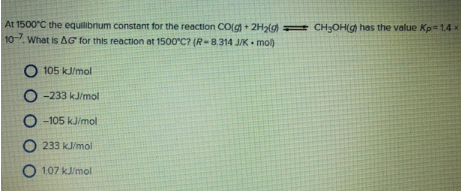# Problem: At 1500 °C the equilibrium constant for the reaction CO(g) + 2H2(g) ⇌ CH3OH(g) has the value Kp = 1.4 x 10 -7. What is Δ G° for this reaction at 1500 °C? (R = 8314 J/K • mol) 105 kJ/mol -233 kJ/mol -105 kJ/mol 233 kJ/mol 1.07 kJ/mol

###### FREE Expert Solution
79% (450 ratings)###### Problem Details

At 1500 °C the equilibrium constant for the reaction CO(g) + 2H2(g) ⇌ CH3OH(g) has the value Kp = 1.4 x 10 -7. What is Δ G° for this reaction at 1500 °C? (R = 8314 J/K • mol)

105 kJ/mol

-233 kJ/mol

-105 kJ/mol

233 kJ/mol

1.07 kJ/mol